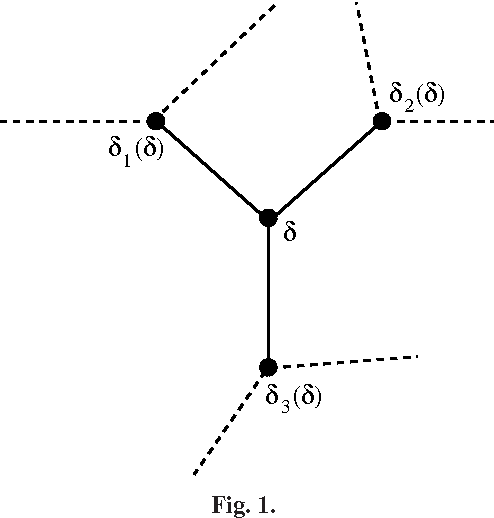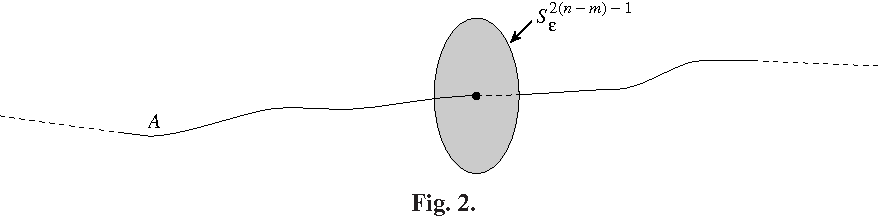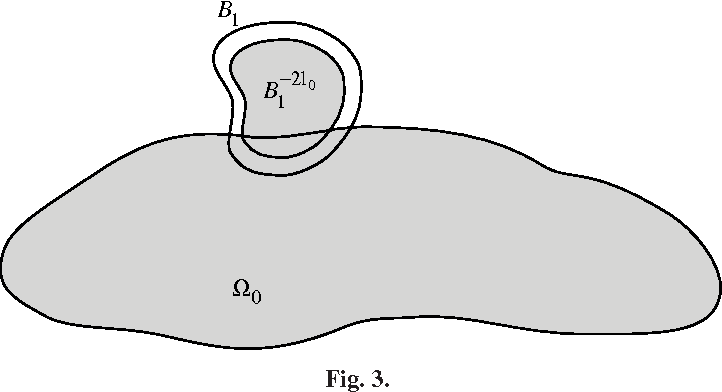Surjunctive group

Known as: Surjunctive
In mathematics, a surjunctive group is a group such that every injective cellular automaton with the group elements as its cells is also surjective… Expand
Wikipedia

Papers overview

Semantic Scholar uses AI to extract papers important to this topic.
2019
2019
Let \$X\$ be a compact metrizable group and \$\Gamma\$ a countable group acting on \$X\$ by continuous group automorphisms. We give… Expand
Is this relevant?
2016
2016
Let \$X\$ be a compact metrizable group and \$\Gamma\$ a countable group acting on \$X\$ by continuous group automorphisms. We give… Expand
Is this relevant?
2014
2014
The category of quasi frames (or qframes) is introduced and studied. In the context of qframes we can jointly study problems… Expand
Is this relevant?
2014
2014
A monoid M is called surjunctive if every injective cellular automata with finite alphabet over M is surjective. We show that all… Expand
Is this relevant?
Highly Cited
1999
Highly Cited
1999
The theorem of Ax says that any regular selfmapping of a complex algebraic variety is either surjective or non-injective; this… Expand
•••Is this relevant?